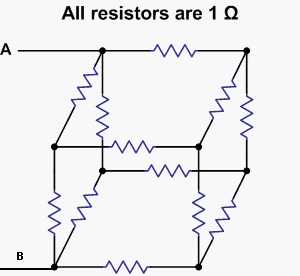# Equivalent Resistance

stardust006how can i solve the equivalent resistance?

I assume R = 2 and I = 3, I know I in node A will be divided. It's just that I don't know what direction I will go to solve for Voltage so I can solve the equivalent resistance.

Is Req = 4/3 Ω ?
on[/b]

Chopin
There are a couple ways you might attack this. One is to set up a system of node-voltage equations like you would for any circuit network. That will guarantee that you can solve it, although it will involve 8 equations in 8 unknowns, so you better have your calculator handy.

The other solution is to try and simplify the network. Since this one is made up entirely of nodes with three edges coming into each one, you might try using Y-Δ transformations on it.

Homework Helper
The network has got a plane of symmetry: The yellow plane in the figure attached. The symmetric nodes (C,D) and (E,F) are at equal potential. The nodes which are at equal potential can be shorted (connected with a wire), it does not change the currents and voltages in the network. Connecting the equivalent nodes, C and D become a single node, and E, F also. Redraw the network.

Req=4/3 Ω is correct.

ehild